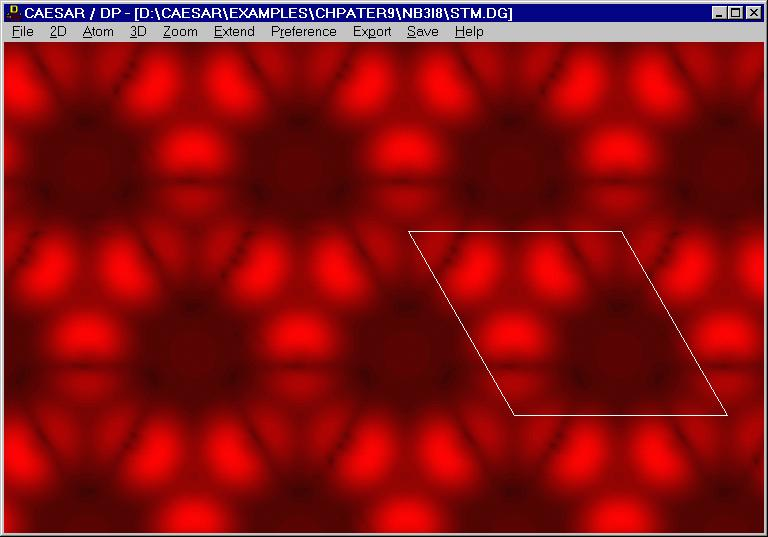# Density of Nb3I8 Layer

β-Nb3X8 (X = Cl, Br, I) is a layered compound. In the Nb-atom sheet of each Nb3X8 layer, the Nb atoms form triangle Nb3 cluster, so that each Nb atom is in a distorted octahedral environment. In each Nb3X8 layer, the top and bottom sheets of X atoms are not equivalent. On the bottom halogen sheet containing that X(2) and X(4) atoms in Figure 9.35 (surface A), the X(4) atoms lie farther away from the Nb-atom sheet than the X(2) atoms (by 0.60, 0.55, and 0.50 Å for X = I, Br and Cl, respectively). On the top halogen sheet containing the X(1) and X(3) atoms in Figure 9.35 (surface B), the X(1) atoms lie farther away from the Nb-atom sheet than the X(3) atoms (by 0.44, 0.33, and 0.35 Å for X = I, Br and Cl, respectively). In principle, the surface of Nb3X8 observed by STM and AFM can be either surface A or surface B.

In the calculated ρ(r0, ef) plot, the density peaks of the three I(2) atoms within a unit cell are closer to one another than expected on the basis of the crystal structure. This effect is caused by the hybridization of the I(2) atom p-orbitals in the half-filled band, and it explains the observation of triangular bright spots in the STM image.Figure. ρ(r0, ef) plot calculated for surface A of a single Nb3I8 layer of β-Nb3I8.

Go back to The Gallery